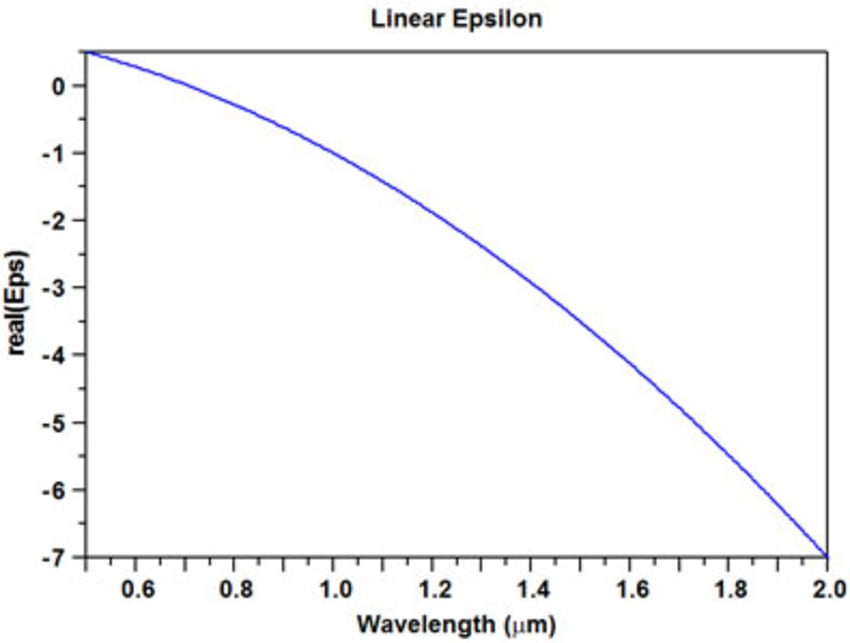# RSoft Application Gallery Note: Negative Index Metamaterials

## Tool Used: FullWAVE

In all known naturally occurring materials, the real part of the index of refraction is positive. However, as shown in reference , this is not a fundamental restriction. It is possible to create negative refraction with left-handed materials – materials whose electrical permittivity and magnetic permeability are simultaneously negative in certain frequency bands. Recently, several artificially structured left-handed meta-materials have been introduced (reference ) and analytical analysis has shown surprising results (references [1,3]). The properties of these new materials are of some considerable interest.

RSoft's FullWAVE, based on the FDTD technique for solving Maxwell's equations, is a very suitable tool for modeling negative index metamaterials: the requirement that ε and μ be simultaneously negative implies a frequency-dependent dispersion model for both properties, which FullWAVE can include with ease. This application note utilizes FullWAVE to investigate the transmission characteristics of a finite Gaussian beam incident through a material with negative refractive index: we present two negative index examples, a negative prism and a negative lens, both with ε = μ = -1. In addition to this application note, please refer to "Tutorial #12 – Negative Refractive Index" in the FullWAVE manual for further details about simulating negative-index metamaterials in FullWAVE.

## Device Layout: Negative Prism

The structure used in this example consists of two components combined to form a simple 2D prism, or wedge, with a negative index of refraction. The material properties of both components are defined by the user-defined material NegIndex (described below), and the background material is air.

The user-defined negative refractive index material NegIndex is defined by a dispersive linear model for ε and μ

D=ϵ(w)E

B=μ(w)H

In this example, we define the wavelength of the incident Gaussian beam as λ0 = 1μm, and ε(w) = μ(w) = 1 + (2 * w2)/(-w02), where w0 = 2π/λ0 is the incident beam frequency. Note that at λ = λ0 = 1μm, ε= μ=-1## Simulation: Negative PrismSimulation results for (left) a prism with a negative refractive index as defined by the user-defined material NegIndex  and (right) a prism with a "normal" refractive index (ε = 2.2 and μ=0). The width of the Gaussian beam is 2μm.

As expected, the refraction angle for the negative index case is tilted to the opposite side than that of the positive index material. Further comparison between the two results reveals some interesting phenomena. In the positive index case the Gaussian beam disperses as expected after leaving the prism, while in the negative index case the field is focused after leaving the prism. Also note that the special case of ε = μ = -1 represents a perfect impedance match (Z = +1) with free space, and for all incident angles, the field pattern will exhibit minimal reflection at the interfaces.

## Device Layout: Negative Concave Lens

This example will demonstrate the propagation of light through a concave lens composed of negative index material. The lens's material properties have been set by the user-defined material NegIndex (described in the previous section), and the background material is air. As in the previous case, the launch has a Gaussian profile and a wavelength of 1 μm.

## Simulation Results: Negative Lens

As expected, the field is clearly focused by the negative concave lens but not by the positive lens.Simulation results for (left) a lens with a negative refractive index as defined by the user-defined material NegIndex, and (right) a lens with a "normal" refractive index (ε = 3 and μ=0). The width of the Gaussian beam is 4 μm.

## References:

 V. G. Veselago "The electrodynamics of substances with simultaneously negative values of ε and μ" Soviet Physics USPEKI 10, 509 (1968).
 D. R. Smith, Willie J. Padilla, D. C. Vier, S. C. Nemat-Nasser, S. Schultz, "A composite medium with simultaneously negative permeability and permittivity," Phys. Rev. Lett. 84, 4184 (2000).
 J. B. Pendry, "Negative refraction makes a perfect lens," Phys. Rev. Lett. 85, 3966 (2000).